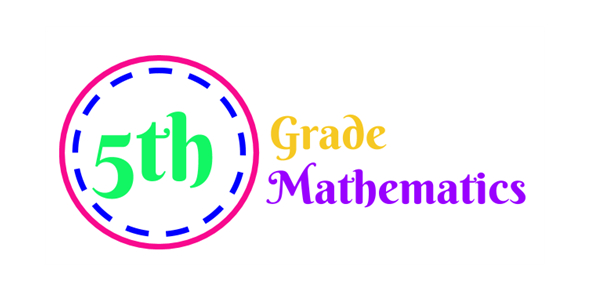# Class 5th Grade Mathematics Quiz!

10 Questions | Attempts: 52832SettingsDo you like mathematics? Our " Class 5th Grade Mathematics Quiz " is here to test your knowledge! Please look at the problems and answer them using multiple-choice. Just test how much time you will take to add the numbers. This is basic addition. You can also play this quiz with your friends for a fun learning time! So let's try out this quiz. We wish you all the best with this quiz!

• 1.
Find the sum of the two numbers? 45678 + 56789 =
• A.

102556

• B.

107657

• C.

105789

• D.

102467

• 2.
Find the sum of the two numbers? 2563 + 5489 =
• A.

8052

• B.

8056

• C.

8096

• D.

8074

• 3.
Find the sum of the two numbers? 9875 + 9624 =
• A.

19499

• B.

19453

• C.

19477

• D.

19563

• 4.
Find the sum of the two numbers? 2356 + 4578 =
• A.

6999

• B.

6986

• C.

6934

• D.

6953

• 5.
Find the sum of the two numbers? 7412 + 3652 =
• A.

11065

• B.

11064

• C.

11061

• D.

11068

• 6.
Find the sum of the two numbers? 5241 + 3245 =
• A.

8486

• B.

8422

• C.

8487

• D.

8489

• 7.
Find the sum of the two numbers? 4125 + 5632 =
• A.

9742

• B.

9757

• C.

9875

• D.

9632

• 8.
Find the sum of the two numbers? 1478 + 9632 =
• A.

11110

• B.

11111

• C.

11123

• D.

11165

• 9.
Find the sum of the two numbers? 6548 + 6987 =
• A.

13535

• B.

13524

• C.

13565

• D.

13874

• 10.
Find the sum of the two numbers? 5845 + 9874 =
• A.

15785

• B.

15719

• C.

15325

• D.

15796

## Related TopicsBack to top
×

Wait!
Here's an interesting quiz for you.